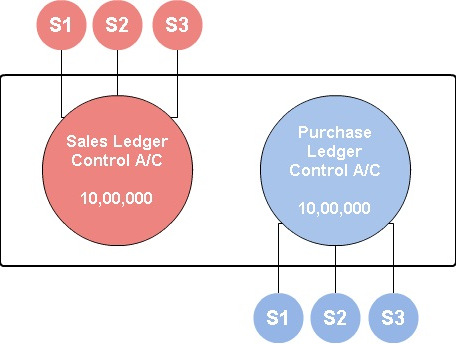# What is Control Account?

## Control Account

An account which has a balance equal to the total of its underlying subsidiary ledger accounts is called a Control Account. These are accounts shown in the general ledger and they serve the purpose of checking if the total in the general ledger is equal to the total of its associated subledgers.

It is a summarized form of all related subledger accounts.

Example

Subledger 1 + Subledger 2 + Subledger 3 +  = Control A/C

In below image, rectangle shows General Ledger, the two big circles are control accounts and the smaller circles depict its respective subledgers, the aggregate of all subledgers is equal to the net amount of its respective control account.

Assuming balances, S1 = 2,00,000, S2 = 3,00,000, S3 = 5,00,000

S1 + S2 + S3 = 10,00,000 (To be shown in GL)## What is the Use of a Control Account?

• It helps to check accuracy between the total of all subledger accounts and its related account in General Ledger.
• It helps to keep the General Ledger free from all redundant information required to prepare financial statements of a business. For example, if we talk about a sales ledger control account, a company can have hundreds and thousands of debtors, hence it will not be practical to have all of them listed in GL.

###### Subscribe
* indicates required